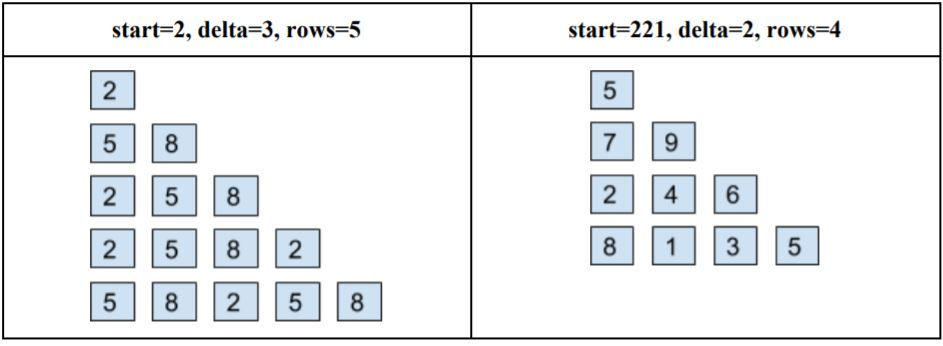# How our teaching methods are unique and different.

Post at 24-02-2023 07:27:58

Our educationist follow a unique approach while teaching by doing the following:
1) Questions sets are explained in an easy flow.
2) Unique strategies in modules for easy and effective learning.
3) Test cases are provided to students for the learning approach.
4) Step by step images for better understanding.
5) Easy and uncomplicated methods to solve problems.

The approaches are designed to help students break all the classroom straightforward textbook learning.

Students can grab and learn all the concepts easily.

As a tremendous platform, our teaching methods are unique and different. We provide a shortcut and quick based recovery approach that helps students recall concepts quickly.

Our solutions are unique and simple from others and nowhere near to straight from textbook learning.

Images used below give a fair idea of what the students will see and learn in various courses. Educationists provide a different and easy way to solve the equations in the easiest and uncomplicated methods as mentioned below:

Problem: Construct a Numeral Triangle according to the following rules. You will be given three positive integers: 's', a starting number; 'd', a delta (the amount by which to increase each number in the triangle); and 'r' the number of rows.

1. The first row contains the number s.
2. Each of the next rows has one more number than the previous row.
3. Each number in the triangle is d more than the previous number in the triangle.
4. Before putting a number in the triangle, it is transformed to a single digit. That is, if the number is more than one digit, replace it by the sum of the digits, repeating until the sum is one digit (for example, 1938 ⇒ 21 ⇒ 3).

Here are two examples of Numeral Triangles:Key points to keep in mind while solving problems are:

5 Steps to Solving a Problem

• Identify the Problem: Sometimes kids will identify one thing as the problem, but it really turns out to be something else. Make sure you understand the situation and clarify the specific problem.
• Generate Ideas: Generate several ideas for solving an issue. Not all of them will work, but you're not trying to pick out ones that will and won't work during this step. Just generate as many ideas as you can.
• Evaluate Ideas: Go through and figure out which ideas are ones to try and which ones to leave behind.
• Decide on a Solution and Try it Pick an idea for solving the problem and give it a try.
• Did it Work? After you've tried to solve the problem, check in to see if it worked. If it did, awesome! If it didn't, just go back and pick another solution that you thought of during step 3.

• 1312 Views